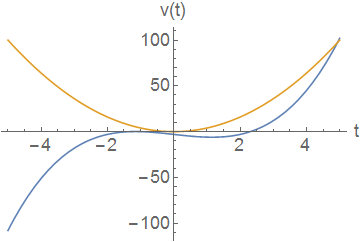# Comparing Velocity and Acceleration 1

The below plot gives two curves representing velocity as a function of time for two different particles.Two curves giving velocity as a function of time of two particles

For which curve is the magnitude of the acceleration greater at $t=0?$

×